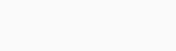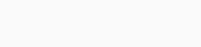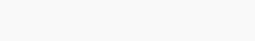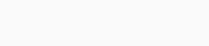## Physics

Question 1.
Answer the following questions regarding earth’s magnetism:
(a) A vector needs three quantities for its specification. Name the three independent quantities conventionally used to specify the earth’s magnetic field.
(b) The angle of dip at a location in southern India is about 18°. Would you expect a greater or smaller dip angle in Britain?
(c) If you made a map of magnetic field lines at Melbourne in Australia, would the lines seem to go into the ground or come out of the ground?
(d) In which direction would a compass free to move in the vertical plane point to, if located right on the geomagnetic north or south pole?
(e) The earth’s field, it is claimed, roughly approximates the field due to a dipole of magnetic moment 8 × 1022 J T-1 located at its centre. Check the order of magnitude of this number in some way.
(f) Geologists claim that besides the main magnetic N-S poles, there are several local poles on the earth’s surface oriented in different directions. How is such a thing possible at all?
Solution:
(a) Angle of declination (magnetic declamatory) θ, angle of dip δ, horizontal component of earth’s magnetic field BH are the quantities which are considered as elements of earth’s magnetic field.
(b) Britain is closer to magnetic north pole hence angle of dip is much larger, nearly 70° in Britain.
(c) Melbourne is closer to south pole, so north of the assumed magnet buried within earth lies inside, hence the field lines would seem to be coming out of the ground.
(d) At geomagnetic north or south pole, angle of dip is 90°, where horizontal component of earth’s magnetic field BH is zero. A compass needle can only turn in horizontal plane, so it can point in any direction as BH = 0, which governs its direction.
(e) Let us consider the magnetic field on surface of earth due to assumed bar magnet of dipole moment 8 × 1022 J T-1 located at centre of earth. The magnetic field at point P equatorial position on earth can be calculated as(f) Localised magnetic dipoles can develop due to magnetised mineral deposits or movement of charged ions in atmosphere.

Question 2.
(a) The earth’s magnetic field varies from point to point in space. Does it also change with time? If so, on what time scale does it change appreciably?
(b) The earth’s core is known to contain iron. Yet geologists do not regard this as a source of the earth’s magnetism. Why?
(c) The charged currents in the outer conducting regions of the earth’s core are thought to be responsible for earth’s magnetism. What might be the battery (i.e., the source of energy) to sustain these currents?
(d) The earth may have even reversed the direction of its field several times during its history of 4 to 5 billion years. How can geologists know about the earth’s field in such distant past?
(e) The earth’s field departs from its dipole shape substantially at large distances (greater than about 30000 km). What agencies may be responsible for this distortion?
(f) Interstellar space has an extremely weak magnetic field of the order of 10-12 T. Can such a weak field be of any significant consequence? Explain.
Solution:
(a) Yes, earth’s field undergoes a change with time. For e×ample, daily changes, annual changes, secular changes with period of the order of 960 years and irregular changes like magnetic storms. Time .scale for appreciable change is roughly a few hundred years.
(b) The earth’s core does contain iron but in the molten form only. This is not ferromagnetic and hence it cannot be treated as a source of earth’s magnetism.
(c) One of the possibilities is the radioactivity in the interior of the earth. But it is not certain.
(d) Earth’s magnetic field gets recorded weakly in certain rocks during their solidification. An analysis of these rocks may reveal the history of earth’s magnetism. The earth’s magnetic field gets modified by the field produced by motion of ions in earth’s ionosphere.
(f) When a charged particle moves in a magnetic field, it is deflected along a circular path such thatwhen B is low, R is high i.e. radius of curvature of path is very large. Therefore, over the gigantic interstellar distance, the deflection of charged particles becomes less noticeable.

Question 3.

A short bar magnet placed with its axis at 30° with a uniform external magnetic field of 0.25 T experiences a torque of magnitude equal to 4.5 × 10-2 J. What is the magnitude of magnetic moment of the magnet?
Solution:
Torque x = MB sinθ 4.5 × 10-2 = M (0.25 sin 30°) Magnetic dipole moment, M = 0.36 J T-1.Question 4.
A short bar magnet of magnetic moment M = 0.32 J T-1 is placed in a uniform magnetic field of 0.15 T. If the bar is free to rotate in the plane of the field, which orientation would correspond to its (a) stable, and (b) unstable equilibrium? What is the potential energy of the magnet in each case?
Solution:
(a) An equilibrium is stable, if on disturbing the magnet, it comes back to same initial state. Bar magnet is in stable equilibrium at 0 = 0° Potential energy.(b) A bar magnet is in unstable equilibrium, if on disturbing from its position, it further gets disturb and do not come back to previous position of equilibrium. At 0 = 180°, the equilibrium is unstable. Potential energyQuestion 5.
A closely wound solenoid of 800 turns and area of cross section 2.5 × 10-4 m2 carries a current of 3.0 A. Explain the sense in which the solenoid acts like a bar magnet. What is its associated magnetic moment?
Solution:A current carrying closely wound solenoid acts like bar magnet. Each of the turn provide a dip’ole moment and all turns together provides the dipole moment of the magnet. Total magnetic moment, M = NIA = 800 × 3 × 2.5 × 10-4 = 0.6 A m2

Question 6.
If the solenoid in the previous question is free to turn about the vertical direction and a uniform horizontal magnetic field of 0.25 T is applied, what is the magnitude of torque on the solenoid when its a×is makes an angle of 30° with the direction of applied field?
Solution:
The solenoid behaves as a bar magnetQuestion 7.
A bar magnet of magnetic moment 1.5 J T-1 lies aligned with the direction of a uniform magnetic field of 0.22 T.
(a) What is the amount of work required by an external torque to turn the magnet so as to align its magnetic moment:

• normal to the field direction,
• opposite to the field direction?

(b) What is the torque on the magnet in cases (i) and (ii)?
Solution:
(a) Work required to turn the dipole(b) Torque when 0 = 90°Question 8.
A closely wound solenoid of 2000 turns and area of cross-section 1.6 × 10-4 m2, carrying a current of 4.0 A, is suspended through its centre allowing it to turn in a horizontal plane.
(a) What is the magnetic moment associated with the solenoid?
(b) What is the force and torque on the solenoid if a uniform horizontal magnetic field of 7.5 × 10-2 T is set up at an angle of 30° with the axis of the solenoid?
Solution:
(a) Magnetic moment associated with solenoid
M = NIA = 2000 × 4 × 1.6 × 10-4 = 1.28 A m2
(b) Force on the solenoid will be zero in uniform magnetic field.
Torque x = MB sinθ = 1.28 × 7.5 × 10-2 × sin 30° or × = 4.8 × 10-2 N m The torque tends to align the solenoid in the direction of magnetic field.

Question 9.
A circular coil of 16 turns and radius 10 cm carrying a current of 0.75 A rests with its plane normal to an external field of magnitude 5.0 × 10-2 T.The coil is free to turn about an a×is in its plane perpendicular to the field direction. When the coil is turned slightly and released, it oscillates about its stable equilibrium with a frequency of 2.0 s-1. What is the moment of inertia of the coil about its axis of rotation?
Solution:
The circular coil carries a dipole momentQuestion 10.
A magnetic needle free to rotate in a vertical plane parallel to the magnetic meridian has its north tip pointing down at 22° with the horizontal. The horizontal component of the earth’s magnetic field at the place is known to be 0.35 G. Determine the magnitude of the earth’s magnetic field at the place.
Solution:
North tip is pointing down at 22° with horizontal, hence the location is in northern hemisphere.Question 11.
At a certain location in Africa, a compass points 12° west of the geographic north. The north tip of the magnetic needle of a dip circle placed in the plane of magnetic meridian points 60° above the horizontal. The horizontal component of the earth’s field is measured to be 0.16 G. Specify the direction and magnitude of the earth’s field at the location.
Solution:
Compass needle points 12° west of geographical north, hence angle of declination 0 is 12° west. North tip of magnetic needle is 60° above horizontal, hence the location is in southern hemisphere and angle of dip is 60°. Magnitude of net magnetic field can be calculated asQuestion 12.
A short bar magnet has a magnetic moment of 0.48 J T-1. Give the direction and magnitude of the magnetic field produced by the magnet at a distance of 10 cm from the centre of the magnet on (a) the axis, (b) the equatorial lines (normal bisector) of the magnet.
Solution:

Question 13.
A short bar magnet placed in a horizontal plane has its a×is aligned along the magnetic north-south direction. Null points are found on the axis of the magnet at 14 cm from the centre of the magnet. The earth’s magnetic field at the place is 0.36 G and the angle of dip is zero. What is the total magnetic field on the normal bisector of the magnet at the same distance as the null-point (i.e., 14 cm) from the centre of the magnet? (At null points, field due to a magnet is equal and opposite to the horizontal component of earth’s magnetic field.)
Solution:
Angle of dip is zero at the place, so earth’s magnetic field is uniform with magnitude 0.36 G in the direction geographi¬cal south to geographical north.Question 14.
If the bar magnet in the previous problem is turned around by 180°, where will the new null points be located? wairii When the bar magnet is turned through 180°, neutral points would lie on equatorial line, so that
Solution:
when the bar magnet is tuned through 180, neutral points would lie on equatorial line, so that

Question 15.
A short bar magnet of magnetic moment 5.25 × 10-2 J T-1 is placed with its a×is perpendicular to the earth’s field direction. At what distance from the centre of the magnet, the resultant field is inclined at 45° with earth’s field on
(a) its normal bisector and
(b) its a×is. Magnitude of the earth’s field at the place is given to be 0.42 G. Ignore the length of the magnet in comparison to the distances involved.
Solution:Question 16.

1. Why does a paramagnetic sample display greater magnetisation (for the same magnetising field) when cooled?
2. Why is diamagnetism, in contrast, almost independent of temperature?
3. If a toroid uses bismuth for its core, will the field in the core be (slightly) greater or (slightly) less than when the core is empty?
4. Is the permeability of a ferromagnetic material independent of the magnetic field? If not, is it more for lower or higher fields?
5. Magnetic field lines are always nearly normal to the surface of a ferromagnet at every point. (This fact is analogous to the static electric field lines being normal to the surface of a conductor at every point.) Why?
6. Would the maximum possible magnetisation of a paramagnetic sample be of the same order of magnitude as the magnetisation of a ferromagnet?

Solution:

1. In paramagnetics, the tendency to disrupt the alignment of molecular dipoles with the external magnetising field arising from random thermal motion is reduced at lower temperatures.
2. In diamagnetics, the molecular dipole moments always align in direction opposite to that of external magnetising field, inspite of the internal motion of atoms.
3. As bismuth is diamagnetic, so the field in the toroid with bismuth core will be slightly less than when the core is empty.
4. No, the permeability of a ferromagnetic material is not independent of the magnetic field. It is more at higher fields.
5. As the magnetic permeability p of a ferromagnet is much larger than unity i.e., p » 1, so magnet field lines are always nearly normal to the surface of a ferromagnet at every point.
6. Yes, but for the maximum possible magnetisation of paramagnetic sample, impractically very high magnetising fields are required.

Question 17.

1. Explain qualitatively on the basis of domain picture the irreversibility in the magnetisation curve of a ferromagnet.
2. The hysteresis loop of a soft iron piece has a much smaller area than that of a carbon steel piece. If the material is to go through > repeated cycles of magnetisation, which piece will dissipate greater heat energy?
3. A system displaying a hysteresis loop such as a ferromagnet, is a device for storing memory? E×plain the meaning of this statement.
4. What kind of ferromagnetic material is used for coating magnetic tapes in a ‘cassette player, or for building ‘memory stores’in modern computer?
5. A certain region of space is to be shielded from magnetic fields. Suggest a method.

Solution:

1. In a specimen of a ferromagnets, the atomic dipoles are grouped together in domains. All the dipoles of a domain are aligned in the same direction and have net magnetic moment. In an unmagnetised substance these domains are randomly distributed so that the resultant magnetisation is zero. When the substance is placed in an external magnetic field, these domains align themselves in the direction of the field. Some energy is spent in the process of alignment when the external field is removed, these domains ‘ do not come back into their random positions completely. The substance retains some magnetisation. The energy spent in the process of magnetisation is f’ not fully recovered. The balance of energy is lost as’ heat. This is the basic cause for irreversibility of the magnetisation curve of a ferromagnetic substance.
2. Carbon steel piece, because the heat produced in complete cycle of magnetisation is directly proportional to he area under the hysteresis loop.
3. Magnetisation of a ferromagnet is not a single valued function of the magnetising ‘ field. Its value for a particular field depends both on the magnetising field and on the history of its magnetisation i.e. how many cycles of magnetisation it has gone through etc. So, the value of magnetisation is a record or memory of its cycles of magnetisation. If information bits can be made to correspond to these cycles, the system displaying such a hysteresis loop can act as a device for storing information.
4. Ferrites or ceramics which is specially treated barium iron oxides.
5. By surrounding the region with soft iron rings, as magnetic field lines will be drawn into the rings and the enclosed space becomes free of magnetic field.

Question 18.
A long straight horizontal cable carries a current of 2.5 A in the direction 10° south of west to 10° north df east. The magnetic meridian of the place happens to be 10° west of ” the geographic meridian.The earth’s magnetic dip is zero. Locate the line of neutral points (ignore the thickness of the cable). (At neutral points, magnetic field due to a current-carrying cable is equal and opposite to the horizontal component of earth’s magnetic field.)
Solution:The neutral point can be achieved at a location above cable, where magnetic field of cable is balanced by earth’s magnetic field BH.Question 19.
A telephone cable at a place has four long straight horizontal wires carrying a current of 1.0 A in the same direction east to west. The earth’s magnetic field at the place is 0.39 G, and the angle of- dip is 35°. The magnetic declination is nearly zero. What are the resultant magnetic fields at point 4.0 cm below the cable?
Solution:
Let us first decide the directions which can best represent the situation.Telephone cable carry a total current of 4.0 A in direction east to west. We want resultant magnetic field 4.0 cm below.Question 20.
A compass needle free to turn in a horizontal plane is placed at the centre of a circular coil of 30 turns and radius 12 cm. The coil is in a vertical plane making an angle of 45° with the magnetic meridian when the current in the coil is 0.35 A, the needle points west to east.
(a) Determine the horizontal component of earth’s magnetic field at the location.
(b) The current in the coil is reversed and the coil is rotated about its vertical a×is by an angle of 90° in the anticlockwise sense looking from above. Predict the direction of the needle. Take the magnetic declination at the places to be zero.
Solution:
Here, n = 30, r = 12 cm = 12 × 10-2 m. i = 0.35 A ,H=? As is clear from figure shown the needle can point west to east only when H = B sin 45°(b) When current in coil is reversed and coil is turned through 90° anticlockwise, the direction of needle will reverse (i.e. it will point from east to west). This follows from figure shown.

Question 21.
A magnetic dipole is under the influence of two magnetic fields. The angle between the field directions is 60°, and one of the fields has a magnitude of 1.2 × 10-2 T. If the dipole comes to stable equilibrium at an angle of 15° with this field, what is the magnitude of the other field?
Solution:
The magnetic dipole experiences torque due to both the fields and is in equilibrium.Question 22.
A monoenergetic (18 keV) electron beam initially in the horizontal direction is subjected to a horizontal magnetic field of 0.4 G normal to the initial direction. Estimate the up or dowri deflection of the beam over a distance of 30 cm (me = 9.11 × 10-31 kg). [Note: Data in this exercise are so chosen that the answer will give you an idea of the effect of earth’s magnetic field on the motion of the electron beam from the electron gun to the screen in a TV set.]
Solution:
Kinetic energy of electronVelocity in x direction remains constant, hence time to cross 30 cmA magnetic force F = euB is acting in vertical direction, which provides acceleration in vertical directionQuestion 23.
A sample of paramagnetic salt contains 2.0 × 1024 atomic dipoles each of dipole moment 1.5 × 10-23 J T-1. The sample is placed under a homogeneous magnetic field of 0.64 T, and cooled to a temperature of 4.2 K. The degree of magnetic saturation achieved is equal to 15%. What is the total dipole moment of the sample for a magnetic field of 0.98 T and a temperature of 2.8 K? (Assume Curie’s law)
Solution:
Dipole moment at complete saturationQuestion 24.
A Rowland ring of mean radius 15 cm has 3500 turns of wire wound on a ferromagnetic core of relative permeability 800. What is the magnetic field B in the core for a magnetising current of 1.2 A?
Solution:
Magnetic field B in the coreQuestion 25.
The magnetic moment vectors μ→S and μ→I associated with the intrinsic spin angular momentum §→  and orbital angular momentum I→  respectively, of an electron are predicted by quantum theory (and verified experimentally to a high accuracy) to be given by μ→S = −(em)S→  and μ→I = −(e2m)I→ Which of these relations is in accordance with the result expected classically? Outline the derivation of the classical result.
Solution:
Out of the two relations given, only one is in accordance with classical physics. This iswhere r is the radius of the circular orbit, which the electron of mass m and charge (- e) completes in time T. Divide (i) by (ii),of quantum mechanics.